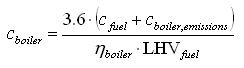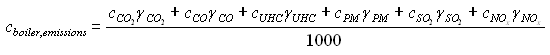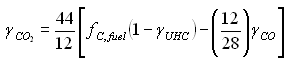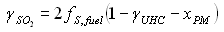# HOMER Grid 1.2

 Navigation: Glossary Boiler Marginal CostType: Intermediate Variable Units: \$/kWh Symbol: cboiler

The boiler marginal cost is the marginal cost of thermal energy from the boiler. HOMER uses this value when calculating the levelized cost of energy. HOMER calculates the boiler marginal cost with the following equation:where: cfuel = cost of fuel [\$/kg of fuel] cboiler,emissions = cost penalty associated with emissions from the boiler [\$/kg of fuel] ηboiler = boiler efficiency [unitless] LHVfuel = the lower heating value of the boiler fuel [MJ/kg]

The factor of 3.6 in the equation above arises because 1 kWh = 3.6 MJ.

Note: The fuel cost in the equation above is per kg, not per L or m3. For fuels denominated in volumetric units, HOMER calculates the cost per kg using the fuel density.

HOMER calculates the cost penalty associated with boiler emissions using the following equation:where: cCO2 = penalty for emissions of CO2 [\$/t] cCO = penalty for emissions of CO [\$/t] cUHC = penalty for emissions of unburned hydrocarbons (UHC) [\$/t] cPM = penalty for emissions of particulate matter (PM) [\$/t] cSO2 = penalty for emissions of SO2 [\$/t] cNOx = penalty for emissions of NOX [\$/t] γCO2 = boiler's carbon dioxide emissions coefficient [kg CO2 / kg fuel] γCO = boiler's carbon monoxide emissions coefficient [kg CO / kg fuel] γUHC = boiler's unburned hydrocarbons emissions coefficient [kg UHC / kg fuel] γPM = boiler's particulate matter emissions coefficient [kg PM / kg fuel] γSO2 = boiler's SO2 emissions coefficient [kg SO2 / kg fuel] γNOx = boiler's NOX emissions coefficient [kg NOX / kg fuel]

HOMER calculates the CO2 emissions coefficient using the following equation:where: fC,fuel = the carbon content of the fuel [unitless] γCO = boiler's carbon monoxide emissions coefficient [kg CO/kg fuel] γUHC = boiler's unburned hydrocarbons emissions coefficient [kg UHC/kg fuel]

The factors of 44/12 and 12/28 in the equation above are used because the molecular weights of C, CO, and CO2 are equal to 12, 28, and 44, respectively.

HOMER calculates the SO2 emissions coefficient using the following equation:where: fS,fuel = the sulfur content of the fuel [unitless] γUHC = boiler's unburned hydrocarbons emissions coefficient [kg UHC/kg fuel] xPM = the proportion of fuel sulfur converted to particulate matter [unitless]

The factor of 2 in the equation above is used because the molecular weight of SO2 (64) is twice that of S (32).

See also:

Levelized Cost of Energy

The Homer Support site has a searchable knowledgebase and additional support options. HOMER Online contains the latest information on model updates, as well as sample files, resource data, and contact information. ©2017 HOMER Energy, LLC